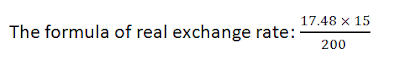# Real Exchange Rate vs Nominal Exchange RateExchange rate means the rate at which one currency will be exchanged for another.
In exchange rate, the words real exchange rate and nominal exchange rate are used while doing transactions in the international market.
The use of both this exchange rate is to buy and sell the currency with the foreign currency in the global market.

## Real exchange rate:

• The real exchange rate is a rate which measures how many times an item of goods purchased locally can be purchased abroad.
• So, it indicates the ratio of items purchased in the domestic market to the items purchased in the foreign market.
• Real exchange rate actually determines the ratio of price in the local market to the price in the foreign market.
• So, it actually indicates the goods and services consumed as compared to another country.
• It is complex and also a difficult method to calculate the real exchange rate.
• So it measures the purchasing power of domestic currency to the foreign currency at a prevailing time.
• Real exchange rate is highly affected by the change in the exchange rate in the global market.

### How to calculate Real exchange rate:

The formula of real exchange rate:For E.g.
If the 1 Kg Price of tea in India is Rs200 and suppose the Price for the same unit in the Dubai is 15 Dhiram. The Exchange rate is 1 Dhiram = Rs17.48: 2.622

## Nominal Exchange Rate:

• Same as the Real exchange rate this exchange rate is also used to buy and sell the goods and services in the international market with another country.
• Nominal exchange rate means a rate by which you can exchange your domestic currency with the foreign currency at any financial institutions like banks, NBFCs etc.
• It is the value of money which is received in an exchange with another currency.
• So in short, the nominal exchange rate is the rate which is presented by the financial institutions.
• If the Nominal exchange rate is high it will benefit an economy a lot in the trading activities. If it is high, the goods and services get more foreign units
• If there is a change in the Exchange rate, Nominal Exchange rate is less affected as compared to the Real exchange rate.

### How to calculate Nominal exchange rate:

There is no particular formula to calculate the Nominal exchange rate. Let’s understand with the help of theory. If you go on a foreign country as it but obvious that if you are in foreign country you need the domestic currency of that country, so you go to the financial institution and demand the foreign currency by giving the Indian currency and the rate at which it exchange is called nominal exchange rate.
For e.g.
Suppose the value of \$1 = INR65, it means that if you go to USA. You will give rs60 and get the \$1 to get the things
• Real exchange rates may be more useful when assessing the impact of exchange rates on international trade than nominal exchange rates as it shows how many times an item of goods can be bought abroad.

Difference between Important Banking Concepts#### What's trending in BankExamsToday

Smart Prep Kit for Banking Exams by Ramandeep Singh - Download here